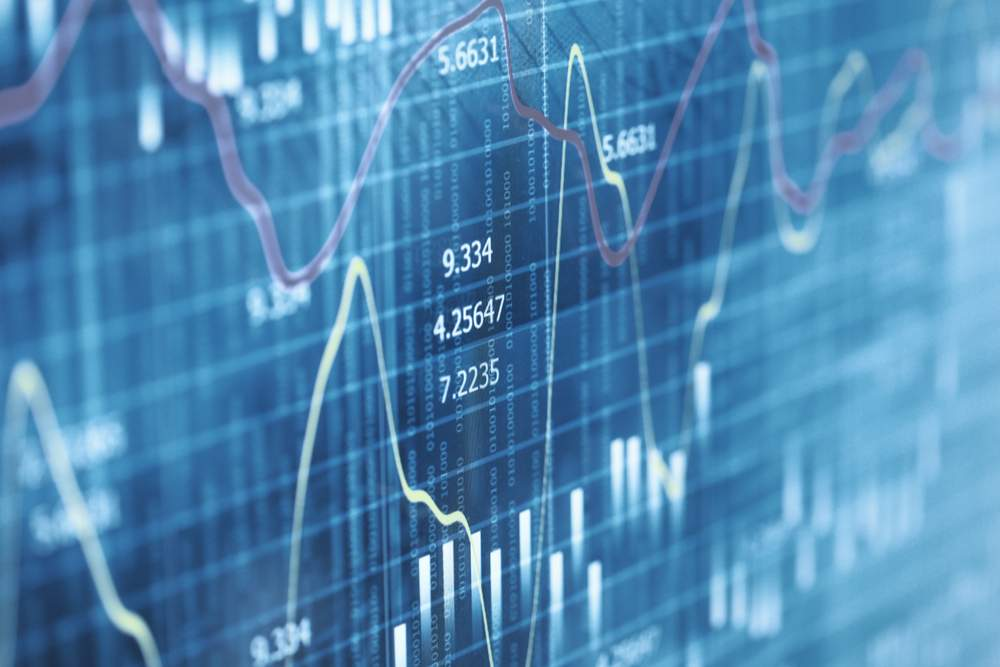# What does PIP stand for in Forex? Price Interest PointPip stands for price interest point as well as a percentage in point. If that definition doesn’t help too much, you’re not alone. While many people, even experienced Forex traders, don’t have a clear understanding of what pips are, they are not hard to figure out.

It is a good idea when trading forex to get answers to the most logical pip trading questions:

1. What is a pip in trading Forex?
2. How are pips calculated?
3. How much is a pip?
4. Do I need a forex pip calculator?

While pip clearly has something to do with interest and points, you will want to know what is a pip and how are pips used when trading FX?

## What is a pip?

A pip is the smallest move in price expressed in the foreign exchange market or said another way, it is simply a unit of measurement used to state the change in value between currencies when there are price changes on an exchange.

Today a pip is not always the last number in a quote. Many brokerage firms can quote prices with an extra decimal point (read on to know more about a pipette). However, a pip is a standard element for price quotes for most trading platforms and millions of traders. That measurement helps traders to be on the same page about financial terms, allowing them to communicate without confusion.

## How much is a pip in trading Forex?

In currency exchange, most pairs are priced out to the fourth decimal place, so one pip looks like this:

• £ 0001

When trading Forex, a pip can be understood as the digit in the fourth place after the decimal point. When you see a currency pair written like this:

• GBO/USD = 1.3102

The 2 is in the fourth decimal place, so that is the pip.

Alright, so now we know a pip of .0001 is the smallest price difference that would be expressed when trading two currencies, right?

Well, yes, unless you count a pipette. With the rising popularity of online trading platforms, traders want more transparency and price competition, while trading currency pairs. That’s why brokerage firms have extended the quote precision to an additional decimal point.

A pipette, or a fractional pip, is just like a pip except it is even smaller, stated as the fifth decimal point. Said another way, it is one-tenth of a pip.

• £ 00001 with the 1 is the pipette.

Or in our example above, if the GBP/USD was expressed to the fifth place after the decimal, the 4 would be the pipette:

• GBO/USD = 1.31024, where the 4 is the pipette.

## How to calculate a pip in Forex trading

Let’s see how to calculate a pip in foreign exchange trading. You can use a Forex pip calculator if you have one, but it is easy to figure out the pip value if you have basic multiplication skills.

First, to calculate a pip value simply multiply the size of the pip by the base currency. In our example above, GBP/USD, the pound, the currency on the left, is the base currency while the dollar, on the right in this example, is the counter currency.

If in our example above, if GBP/USD moves from 1.31024 to 31025, that move higher by .00001 USD is movement of one pipette.

To figure out the value per pip movement when trading, simply multiply your position size by the pip, like this:

• Position size x 0.0001 = value of a pip movement
• Position size x 0.00001 = value of a pipette movement

So, for example, if you were trading that GBP/USD, and your position size is 50,000 units, the monetary value of a change in pip would be calculated like this:

• 50,000 (position size) x 0.0001 = \$5 per pip

Here, you would earn \$5 per pip. Of course, a decrease in pip in the example above would cause a trader to lose money by the same rate.

## Calculating Forex pip values with other currencies

To calculate the pip value in Forex when the deposit currency and counter currency are the same is easy as seen above, but when the counter currency and depositing currency are different, the math is a little trickier. However, if you understand all the terms, it is easy to figure out and you likely do not need a Forex pip calculator.

A pip value varies by currency pair. However, it is equal to:

• (One Pip / Exchange Rate) * Lot Size, meaning trade size

The pip value in the GBP/USD currency pair is calculated using the following info:

One Pip: 0.0001

Account Base Currency: GBP

Currency Pair: GBP/USD

Exchange Rate: 1.31024 (GBP/USD)

Lot Size: 1 Lot (100000 USD)

Please keep in mind that a standard lot is 100,000 units of the base currency. So, in our example above, the standard lot size is equal to 100,000 GBP. There are three other typical lot sizes to understand. A mini-lot is equivalent to 10,000 units, a micro-lot is equal to 1000 units and a nano-lot is equal to 100 units.

r1investing is an online broker who prioritizes a deep understanding of Forex as well as transparent conditions for trading. That’s why millions of European traders stay loyal to the broker. With ROI, investors can craft their strategies efficiently. For that, the broker offers: